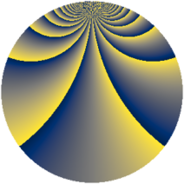# Properties

 Label 1815.2.bpLevel $1815$ Weight $2$ Character orbit 1815.bp Rep. character $\chi_{1815}(41,\cdot)$ Character field $\Q(\zeta_{110})$ Dimension $7040$ Sturm bound $528$

# Related objects

## Defining parameters

 Level: $$N$$ $$=$$ $$1815 = 3 \cdot 5 \cdot 11^{2}$$ Weight: $$k$$ $$=$$ $$2$$ Character orbit: $$[\chi]$$ $$=$$ 1815.bp (of order $$110$$ and degree $$40$$) Character conductor: $$\operatorname{cond}(\chi)$$ $$=$$ $$363$$ Character field: $$\Q(\zeta_{110})$$ Sturm bound: $$528$$

## Dimensions

The following table gives the dimensions of various subspaces of $$M_{2}(1815, [\chi])$$.

Total New Old
Modular forms 10720 7040 3680
Cusp forms 10400 7040 3360
Eisenstein series 320 0 320

## Trace form

 $$7040q - 4q^{3} + 176q^{4} + 10q^{6} + 18q^{9} + O(q^{10})$$ $$7040q - 4q^{3} + 176q^{4} + 10q^{6} + 18q^{9} + 54q^{12} + 28q^{15} + 144q^{16} + 10q^{18} + 60q^{19} + 52q^{22} - 118q^{24} - 176q^{25} - 10q^{27} + 80q^{28} - 64q^{31} + 34q^{33} - 40q^{34} - 34q^{36} - 12q^{37} + 10q^{39} + 42q^{42} + 20q^{46} + 38q^{48} - 140q^{49} - 36q^{51} + 44q^{52} - 154q^{54} - 12q^{55} - 124q^{57} + 24q^{58} - 58q^{60} - 20q^{61} - 50q^{63} + 452q^{64} - 128q^{66} + 88q^{67} - 30q^{69} - 36q^{70} + 120q^{72} - 84q^{73} - 6q^{75} + 396q^{76} + 84q^{78} + 184q^{79} - 18q^{81} - 96q^{82} + 80q^{84} - 132q^{85} + 252q^{88} + 88q^{90} - 72q^{91} + 6q^{93} + 40q^{94} - 10q^{96} + 60q^{97} + 16q^{99} + O(q^{100})$$

## Decomposition of $$S_{2}^{\mathrm{new}}(1815, [\chi])$$ into newform subspaces

The newforms in this space have not yet been added to the LMFDB.

## Decomposition of $$S_{2}^{\mathrm{old}}(1815, [\chi])$$ into lower level spaces

$$S_{2}^{\mathrm{old}}(1815, [\chi]) \cong$$ $$S_{2}^{\mathrm{new}}(363, [\chi])$$$$^{\oplus 2}$$